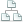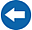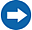Home >Tips >Excel 2016 from Scratch >TRUNC and ROUNDSite map

# Excel 2016 from Scratch Exercise Modify Numbers – the TRUNC and ROUND functions

## An Excel tutorial by Peter KalmstromIn this exercise in the Excel 2016 from Scratch series, Peter Kalmstrom shows how to manipulate numbers with these functions:
• TRUNC: shorten or truncate a number to a set number of decimals without rounding.
• ROUND: round a number to a set number of decimals or to even tens.
Note that these functions really alter the data in your worksheet, so calculations with shortened or rounded numbers will not be exact.

When you want exact calculations but still don't want to see many decimals, use the Excel formatting option that changes how many decimals are displayed without changing the numbers. Peter has used this formatting option in most of his earlier demonstrations.

### Exercise

If you want to try the exercise yourself, please download the Excel file Peter uses in the demo!

### Content

This is what Peter shows in the demo below:

• How to name a cell to use it as an absolute reference.
• How to calculate the absolute value of a price including a discount.
• How to fill a formula to cells below by double clicking.
• How to calculate a shortened value of a price including a discount.
• How to calculate a value of a price including a discount rounded to one decimal.
• How to calculate a value of a price including a discount rounded to even tens.
Peter uses Excel 2016 for his demo, but the Excel calculation functions are the same for earlier versions of Excel.